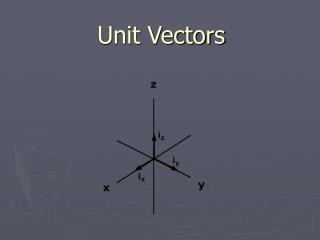Download PresentationUnit VectorsUnit Vectors - PowerPoint PPT Presentation

Download PresentationUnit Vectors
An Image/Link below is provided (as is) to download presentation

Download Policy: Content on the Website is provided to you AS IS for your information and personal use and may not be sold / licensed / shared on other websites without getting consent from its author. While downloading, if for some reason you are not able to download a presentation, the publisher may have deleted the file from their server.

- - - - - - - - - - - - - - - - - - - - - - - - - - - E N D - - - - - - - - - - - - - - - - - - - - - - - - - - -
Presentation Transcript

1. Unit Vectors

2. Old school • Consider vector B, which is expressed as B and θ where B is the magnitude and θ is the direction • We know that the vector has components in the x and y axis (every vector does) Bx and By • We can find these components using trig functions and pythag theorem

3. We always MUST consider the x and y components of a vector to do any type of physics or math problems

4. New School • Vectors can also be expressed in terms of unit vectors • A unit vector is a dimensionless vector having a magnitude of exactly 1 • The unit vector is only used to specify a given direction • i, j, k are used to represent unit vectors pointing in the direction of the positive x, y, z directions

5. If we go back to vector B, now we can express it as B at θ = Bxi + Byj since Bx is in the i direction and By is in the j direction So any position vector R can be written as R = Rxi + Ryj (+ Rzk) Ex. If R = 15 m @ 35o , it can be broken into x=12.29 m and y=8.6 m so… R = 12.29 i + 8.6 j m

6. Adding vectors in unit vector form becomes very easy A + B, where A = Axi + Ayj , B = Bxi + Byj So if A + B = C, then C = (Ax + Bx) i + (Ay + By) j And once we have C, then we can still find it’s polar coordinates, or magnitude and direction (pythag & trig)

7. Sometimes we will do 3 dimensional problems. You can actually still use pythag theorem to find the resultant magnitude, but never anything with angles (at least for us) • You can add (we saw this), subtract (add opposite), and multiply unit vectors, just like before ex. Find 5A where A = Ax i + Ay j 5A = 5Ax i + 5Ay j

8. Find the sum of two vectors a) in unit vector notation and b) polar coordinates if A = 2i + 2j and B = 2i – 4j

9. A particle undergoes three consecutive displacements: d1 = 15i + 30j + 12k cm d2 = 23i – 14j – 5k cm d3 = -13i + 15j cm Find the resultant magnitude of this displacement.